simdata {baycn} R Documentation

## simdata

### Description

A function for simulating data under various topologies for continuous and mixed data.

### Usage

```simdata(
graph = "gn4",
N = 500,
b0 = 0,
ss = 1,
s = 1,
p = 0.6,
q = 0.45,
ssc = 0.2,
nConfounding = 2
)
```

### Arguments

 `graph` A character string of the graph for which data will be simulated. The graphs that can be chosen are m1_ge, m1_gv, m1_cp, m1_cc, m1_iv, m2_ge, m2_gv, m2_cp, m2_cc, m2_iv, m3_gv, m3_cp, m3_cc, m3_iv, mp_ge, mp_gv, gn4, gn5, gn8, gn11, layer, layer_cp, layer_iv, and star. The following figures show the graph for each of the topologies listed above. The nodes with a circle around the name are normally distributed and the nodes with a diamond around the name are distributed multinomial. The nodes labeled with a C represent confounding variables. The Principle of Mendelian Randomization (PMR) can be used on graphs with discrete and continuous random variables. This introduces the constraint that the continuous random variables cannot be parents of discrete random variables and edges between these types of variables only have two states: absent and directed with the discrete random variable as the parent. m1_ge - Topology M1 with three continuous random variables. In this case M1 has two other Markov equivalent graphs. m1_gv - Topology M1 with one discrete random variable U and two continuous random variables. When using the PMR this graph does not have any other Markov equivalent graphs. We consider three types of confounding variables (Yang et al., 2017): m1_cp - Topology M1 with n common parent confounding variables. m1_cc - Topology M1 with n common child confounding variables. m1_iv - Topology M1 with n intermediate confounding variables.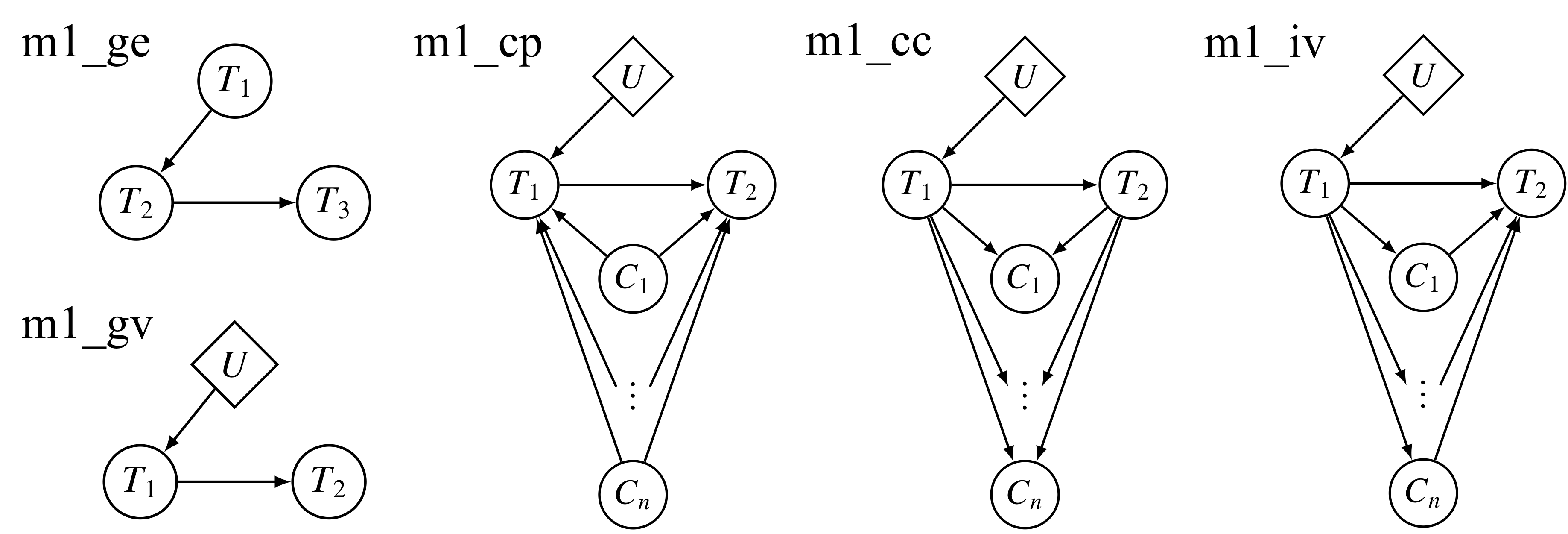m2_ge - Topology M2 with three continuous random variables. This graph is a v structure and does not have any other Markov equivalent graphs. m2_gv - Topolog M2 with one discrete random variable U and two continuous random variables. This graph is a v structure and does not have any other Markov equivalent graphs. m2_cp - Topology M2 with n common parent confounding variables. m2_cc - Topology M2 with n common child confounding variables. m2_iv - Topology M2 with n intermediate confounding variables.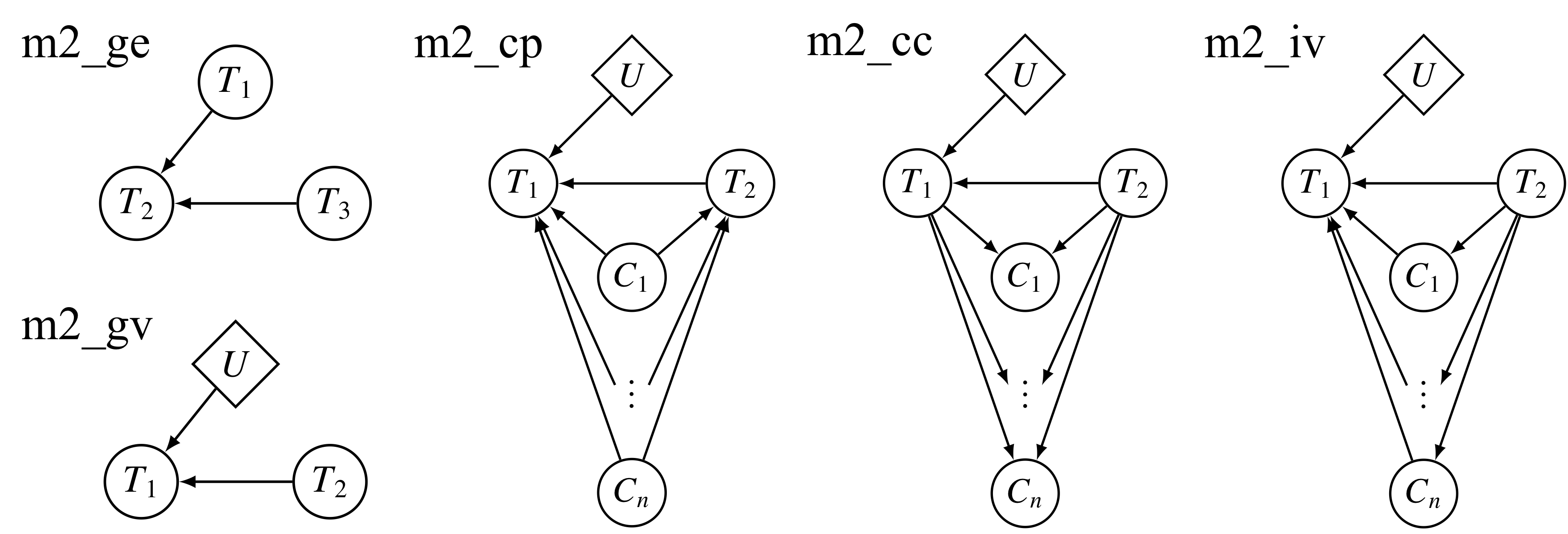m3_gv - Topolog M3 with one discrete random variable U and two continuous random variables. m3_cp - Topology M3 with n common parent confounding variables. m3_cc - Topology M3 with n common child confounding variables. m3_iv - Topology M3 with n intermediate confounding variables.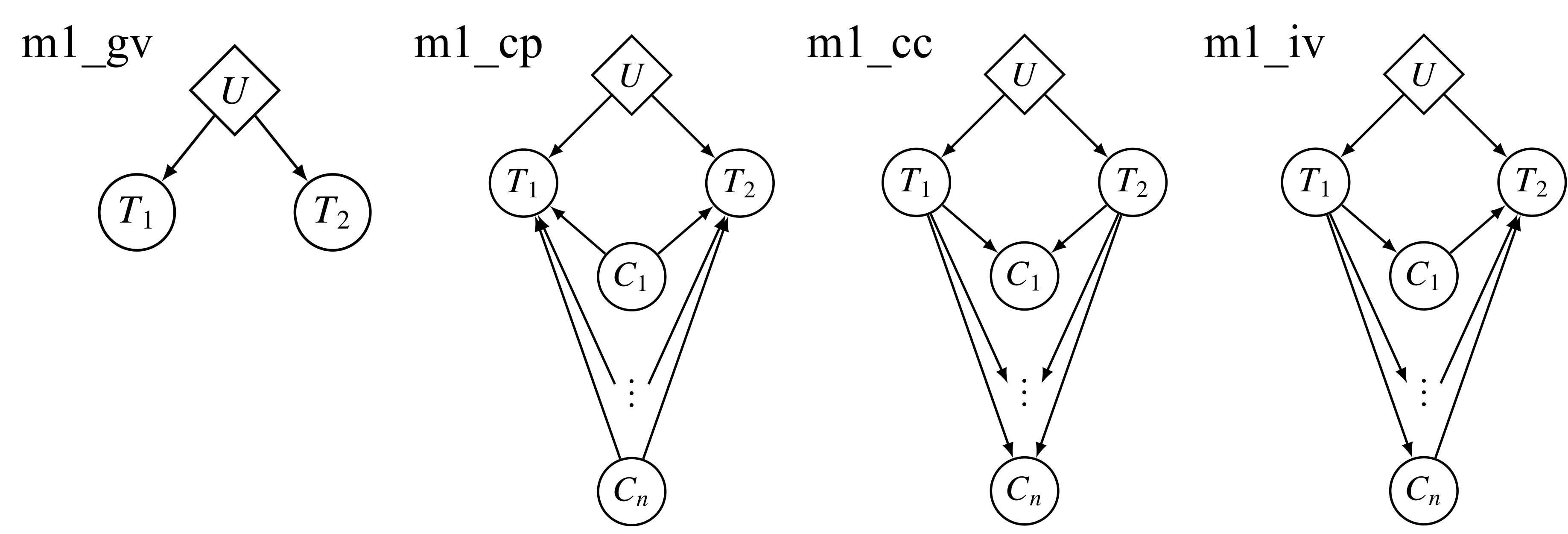mp_ge - The multi-parent topology with continuous random variables. This graph is made up of multiple v structures and has no other Markov equivalent graphs. mp_gv - The multi-parent topology with one discrete random variable. This graph is made up of multiple v structures and has no other Markov equivalent graphs.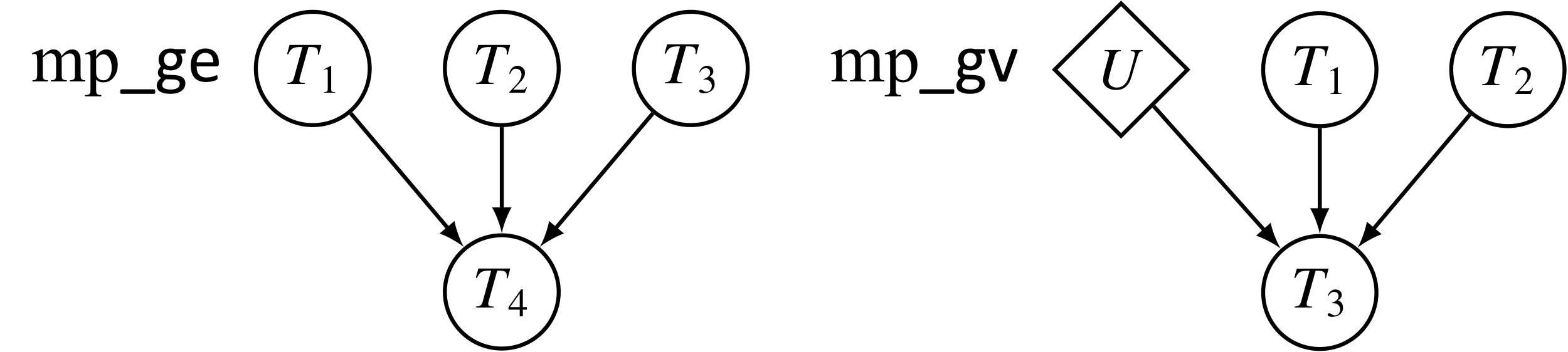gn4 - Topology GN4 is formed by combining topologies M1 and M2. The Markov equivalence class for this topology is made up of three different graphs. gn5 - Topology GN5 has three other Markov equivalent graphs. gn8 - Topology GN8 has three overlapping cycles, two v structures, and two other Markov equivalent graphs. gn11 - Topology GN11 has two sub-graphs separated by a v structure at node T6.layer - The layer topology has no other Markov equivalent graphs when using the PMR and is made up of multiple M1 topologies. layer_cp - The layer topology with 2 common parent confounding variables. layer_iv - The layer topology with 2 intermediate confounding variables.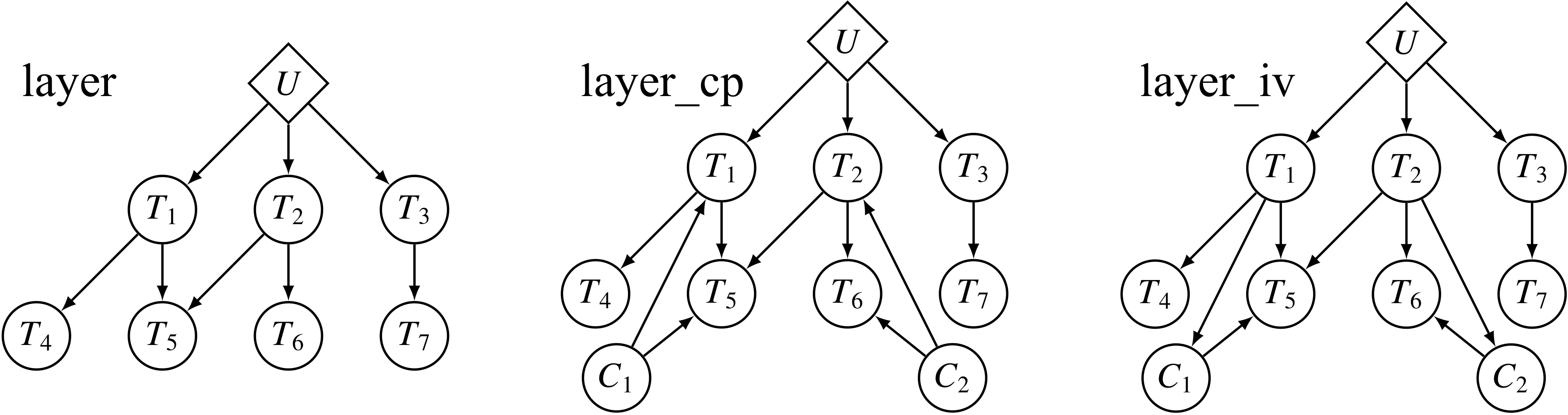star - The star topology has no other Markov equivalent graphs when using the PMR and is made up of multiple M1 topologies.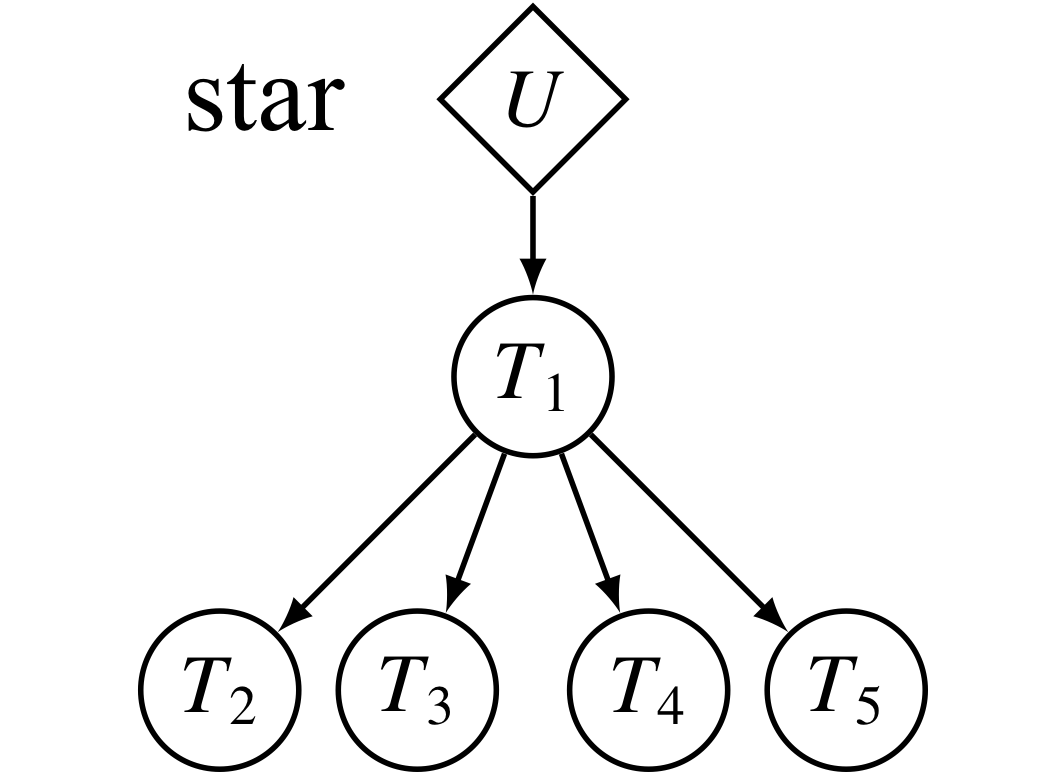`N` The number of observations to simulate. `b0` The mean of the variable if it does not have any parents. If the variable has one or more parents it is the slope in the linear model that is the mean of the normally distributed variables. `ss` The coefficient of the parent nodes (if there are any) in the linear model that is the mean of the normally distributed variables. This coefficient is referred to as the signal strength. `s` The standard deviation of the normal distribution. `p` The probability of success for a binomial random variable. `q` The frequency of the reference allele. `ssc` The signal strength of the confounding variables. `nConfounding` The number of confounding variables to simulate.

### Value

A matrix with the variables across the columns and the observations down the rows.

### References

Yang, F., Wang, J., The GTEx Consortium, Pierce, B. L., and Chen, L. S. (2017). Identifying cis-mediators for trans-eQTLs across many human tissues using genomic mediation analysis. Genome Res. 27, 1859-1871.

### Examples

```# Generate data under topology GN4.
data_gn4 <- simdata(graph = 'gn4',
N = 500,
b0 = 1,
ss = 1,
s = 1)

# Display the first few rows of the data.
data_gn4[1:5, ]

# Generate data under topology M1 with 3 intermediate confounding variables.
data_m1_iv <- simdata(graph = 'm1_iv',
N = 500,
b0 = 0,
ss = 1,
s = 1,
q = 0.1,
ssc = 0.2,
nConfounding = 3)

# Show the first few rows of the data.
data_m1_iv[1:5, ]

```

[Package baycn version 1.2.0 Index]